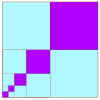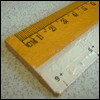Search by Topic

Filter by: Content type:
Age range:
Challenge level:

There are 17 results

Broad Topics > Patterns, Sequences and Structure > Limits of SequencesLitov's Mean Value Theorem

Age 11 to 14 Challenge Level:

Start with two numbers and generate a sequence where the next number is the mean of the last two numbers...Squareness

Age 16 to 18 Challenge Level:

The family of graphs of x^n + y^n =1 (for even n) includes the circle. Why do the graphs look more and more square as n increases?Slide

Age 16 to 18 Challenge Level:

This function involves absolute values. To find the slope on the slide use different equations to define the function in different parts of its domain.Approximating Pi

Age 14 to 18 Challenge Level:

By inscribing a circle in a square and then a square in a circle find an approximation to pi. By using a hexagon, can you improve on the approximation?Climbing Powers

Age 16 to 18 Challenge Level:

$2\wedge 3\wedge 4$ could be $(2^3)^4$ or $2^{(3^4)}$. Does it make any difference? For both definitions, which is bigger: $r\wedge r\wedge r\wedge r\dots$ where the powers of $r$ go on for ever, or. . . .A Swiss Sum

Age 16 to 18 Challenge Level:

Can you use the given image to say something about the sum of an infinite series?Summing Geometric Progressions

Age 14 to 18 Challenge Level:

Watch the video to see how to sum the sequence. Can you adapt the method to sum other sequences?Age 16 to 18 Challenge Level:

Compares the size of functions f(n) for large values of n.Light Blue - Dark Blue

Age 7 to 11 Challenge Level:

Investigate the successive areas of light blue in these diagrams.Ruler

Age 16 to 18 Challenge Level:

The interval 0 - 1 is marked into halves, quarters, eighths ... etc. Vertical lines are drawn at these points, heights depending on positions. What happens as this process goes on indefinitely?Small Steps

Age 16 to 18 Challenge Level:

Two problems about infinite processes where smaller and smaller steps are taken and you have to discover what happens in the limit.Infinite Continued Fractions

Age 16 to 18

In this article we are going to look at infinite continued fractions - continued fractions that do not terminate.Continued Fractions II

Age 16 to 18

In this article we show that every whole number can be written as a continued fraction of the form k/(1+k/(1+k/...)).Continued Fractions I

Age 14 to 18

An article introducing continued fractions with some simple puzzles for the reader.Zooming in on the Squares

Age 7 to 14

Start with a large square, join the midpoints of its sides, you'll see four right angled triangles. Remove these triangles, a second square is left. Repeat the operation. What happens?Archimedes and Numerical Roots

Age 14 to 16 Challenge Level:

The problem is how did Archimedes calculate the lengths of the sides of the polygons which needed him to be able to calculate square roots?Little and Large

Age 16 to 18 Challenge Level:

A point moves around inside a rectangle. What are the least and the greatest values of the sum of the squares of the distances from the vertices?